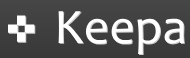## lunedì 20 dicembre 2010

Google Spreadsheet is a powerful and free tool to manage complex spreadsheets. Its advanced features make it a viable alternative to desktop programs like Excel. This tutorial illustrates five simple formulas that help you simplify the way you work with this application.

### Image()

Image() returns a picture from a specific URL contained in a cell or #N/A if the URL doesn’t contain any image. This formula can be useful when you have to check a large quantity of links to verify. The use is very simple: if you want to convert into a picture the URL in A2, select the cell B2 and type the following formula: =IMAGE(A2), where A2 must contain a valid URL. This is the result:GoogleTranslate() help translate text from and into one of over 40 languages using Google Translate. This formula takes 3 parameters: =GoogleTranslate(“text”, “source language”,”target language”). If you want to traslate a text from english to italian use this formula: =GOOGLETRANSLATE(A2, “en”,”it”). This is the result:### Split()

This formula splits a string based on the given delimiter (for example “;”, “,”, “|”, “-”,…) and put each section into a separate column in the row. This formula gives two parameter as input: the string you want to split and the separator. For example if you need to split the string in the cell A2 you can use this formula: =SPLIT(A2; “;”) and this is the result:Hyperlink merge an URL address and a string into a clickable hyperlink. This function gives in input two parameters: =hyperlink(“URL”,”Title”). If you want to merge into a hyperlink A2 and B2 use this code in B3: =HYPERLINK(A2, B2). This is the result:### Days360()

Days360() returs the difference between two dates. If you want to show the difference in days between A2 and B2 use this formula: =DAYS360(A2,B2) and this is the result:"

### Keepa: un ottimo strumento per tracciare i prezzi su Amazon

Keepa è un sito web e un'estensione per browser che consente di tracciare lo storico dei prezzi dei prodotti su Amazon. È uno strumento ...